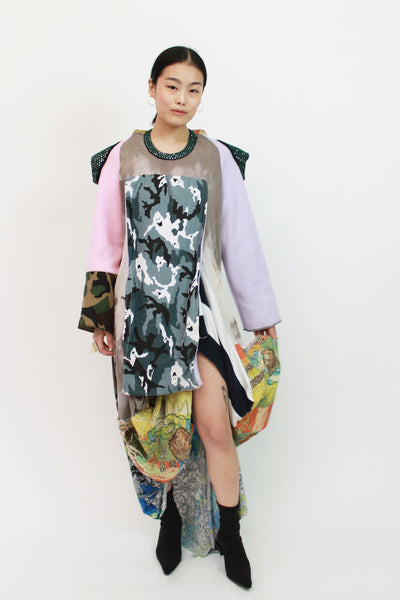# R2W 2.0 FEMAILED Long dress sample 2

FEMAIL

\$ 413.00

T          2 layers X 50.00                             = \$100.00

MS                                                             = \$220.00

C colledged camo (2) X 10%(T)    = \$20.00

Pieces from Aunty K (3) X 10%(T)    = \$30.00

Top from Callen (4) X 10%(T)   = \$40.00

Top from Gma (3) X 10%(T)   = \$30.00

J collage textile/C table cloth (10) X 10%(T)   = \$100.00

MH                                                             = \$38.00

Chinese printed dress  = \$8.00

Fleece  = \$10.00

Ghost text  = \$15.00

Blue knit  = \$5.00

L                                                                 =\$30.00

Camilla    = \$15.00

Janelle     = \$15.00

T(\$100.00)+M(MS(\$220.00)+MH(\$38.00))+L(\$15.00+\$15.00) = 413.00

More from this collection